# Astro 122: Astronomy of Stars and Galaxies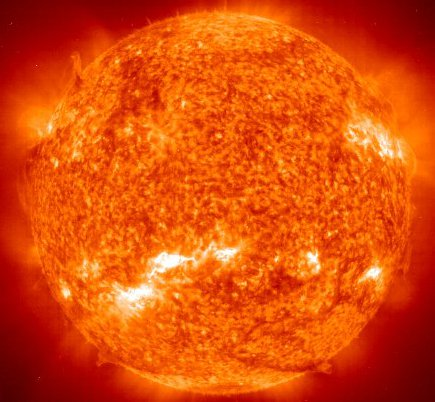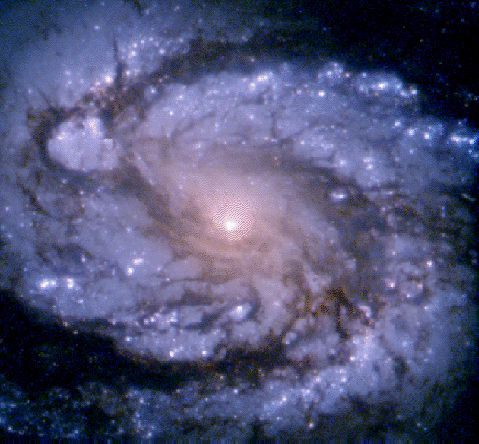# Introduction: Methodology

• ### Establishing observed relations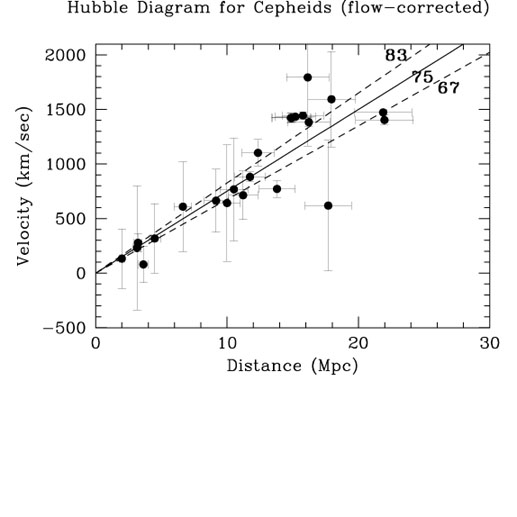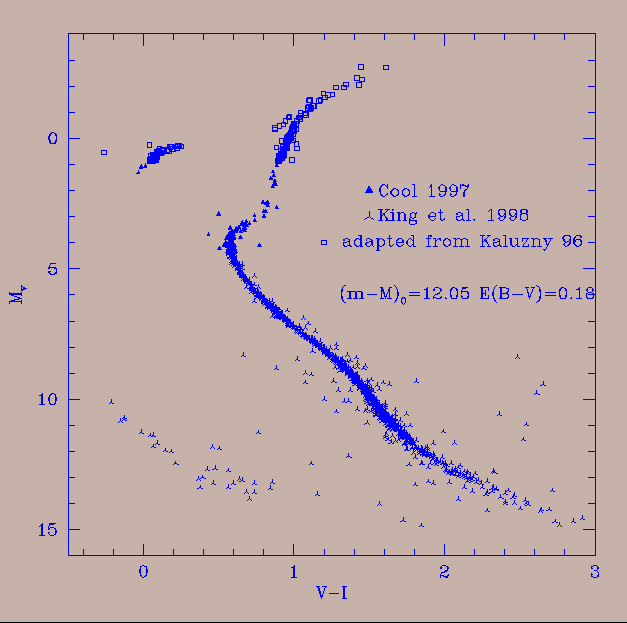• ### Developing theories based on Physics that explain observations. Astronomy becomes Astrophysics

• At the heart - "Copernicus Principle" - we assume that the laws of physics are the same everywhere. Best support - atomic spectra from distant galaxies.
• Physical theories are formulated using math. Math is the most concise language to express relations between things. It also provides tool to deduce new results.

# Lecture 1: Distances in Astronomy

## Sizes of things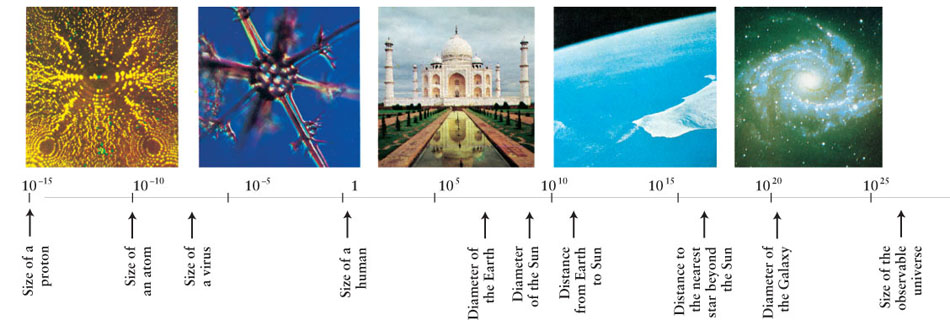## Powers of 10

When dealing with quantities (distances, sizes, masses ...) that can take a vast range of values, we use 'powers of 10' notation.

### For example, distances in meters:

100 m = 10*10 m = 102 m
1000 m = 10*10*10 m = 103 m = 1 km (kilo)
1000000 m = 106 m = 1 Mm (Mega)
1000000000 m = 109 m = 1 Gm (Giga)

### Small quantities are divided by powers of ten, giving negative powers

0.01 m = 1/(10*10) m = 1/(102)m = 10-2 m = 1 cm (centi)
0.001 m = 1/1000 m = 1/(103)m = 10-3 m = 1 mm (milli)
0.000001 m = 1/1000000 m = 1/(106)m = 10-6 m = 1 micron
0.000000001 m = 1/1000000000 m = 1/(109)m = 10-9 m = 1 nm (nano)

## Manipulating Powers of 10

### how to write uneven numbers in powers of 10 ?

as the product of two terms:     13586 = 1.3568*104

### Powers of 10 are easy to multiply

(1.357*104) * (0.962*1035) = (1.357*0.962)* (104*1035) = 1.305*104+35 = 1.305*1039

### but not to add !

1.3568*104 + 0.962*1035 = ??         (1.3568*10-31 + 0.962)*1035

### We say 'of order' when we speak aproximately, only as accurately as the power of 10.

Distance 1.3568*104 m is 'of order' of 104 m.     What about 9.6*1012 ?

## Light units for distances

Light travels at the speed:
speed of light = c = 2.9979 x 108 m/s

Define new units of length based on speed of light:

light-second = distance travelled by light in one second = 3 x 108 m = 3 x 105 km = 300,000 km

light-year = ly = distance travelled by light in one year = 9.46 x 1015 m = 9.46 x 1012 km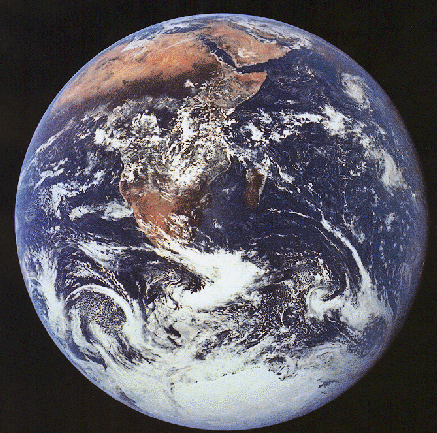## The Earth

• Radius = 6,378 km
• Diameter = 12,756 km
• Circumference = 40,000 km

Light could travel around the Earth's equator about 8 times in one second.

## The Earth seen from the Moon

• Distance between the Earth and the Moon is
3.84 x 105 km.
• Light takes 1.3 seconds to travel from the Earth to the Moon.
• Distance between the Earth and the Moon is about
30 x larger than the Earth's diameter.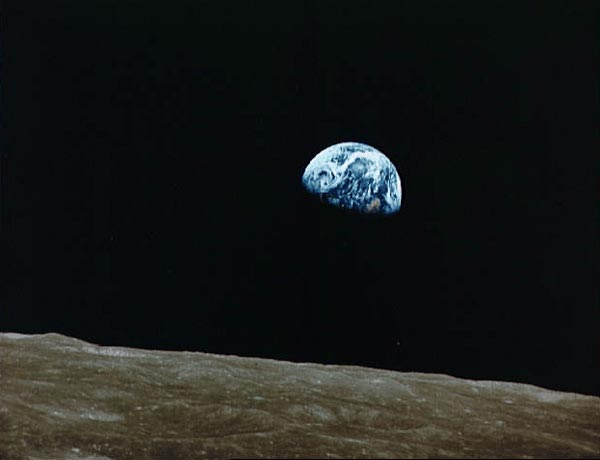## The Sun

• The Sun's radius = 6.96 x 108 m
= 6.96 x 105 km
• The Sun's diameter = 1.39 x 106 km
= 4.6 light-seconds
• The Sun is about 100 x larger in radius than the Earth.
• The Sun's radius is about 1.8 x larger than the distance between the Earth and the Moon.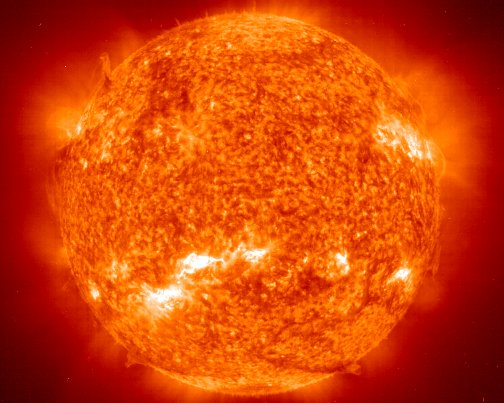## The Earth's Orbit Around the Sun

• Distance between the Sun and the Earth
= 1.5 x 1011 m
= 1.5 x 108 km
= 500 light-seconds
= 8.3 light-minutes
• An Astronomical Unit (AU) is defined to be the average distance between the Sun and the Earth.
• 1 AU is about 100 x larger than the Sun's diameter.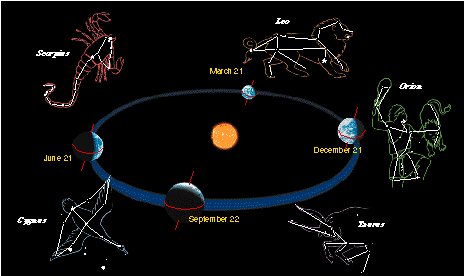## Other "Nearby" Distances:

Average distance between the Sun and Pluto = 40 AU = 5.5 light-hours

Closest star system to the Sun is Alpha Centauri.
Distance between the Sun and Alpha Centauri = 300,000 AU = 4.1 light-years

## The Pleiades Star Cluster

• The Pleiades is a cluster of over 3000 stars.
• Cluster is about 13 ly across
• Cluster is about 400 ly away from us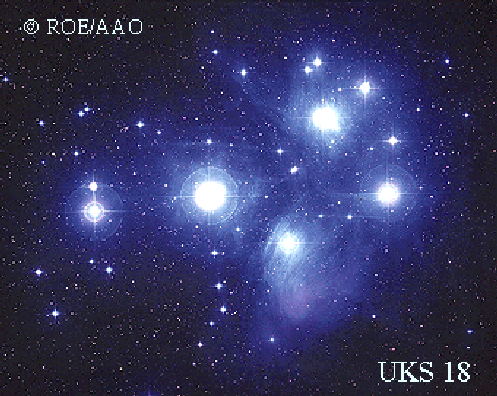## The Milky Way Galaxy

• Our Sun is not at the centre of the Milky Way!
• Distance from Sun to Galactic Centre = 2.8 x 104 ly
• Diameter of Milky Way's disk
= 105 ly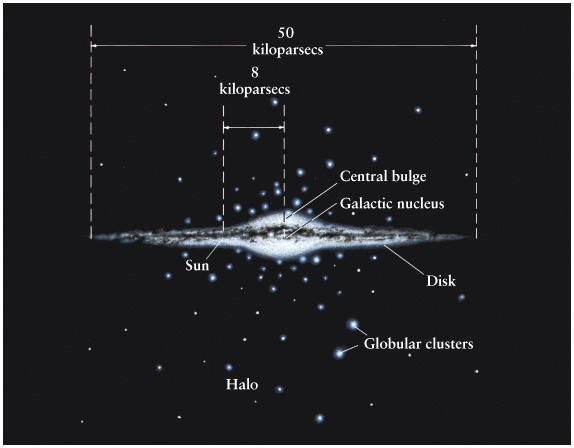## The Andromeda Galaxy

• Andromeda is the nearest big spiral galaxy similar to the Milky Way.
• Distance from the Milky Way to Andromeda = 2 x 106 ly
• Andromeda and the Milky Way are the two largest galaxies in the Local Group of about 30 galaxies.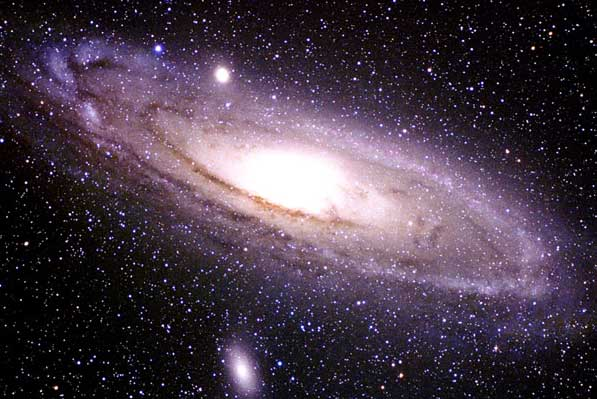## The Virgo Cluster of Galaxies

• The Virgo Cluster is the closest cluster of galaxies, with about 2000 member galaxies.
• Distance from the Local Group to the Virgo Cluster = 6 x 107 ly
• Size of the Virgo Cluster = 107 ly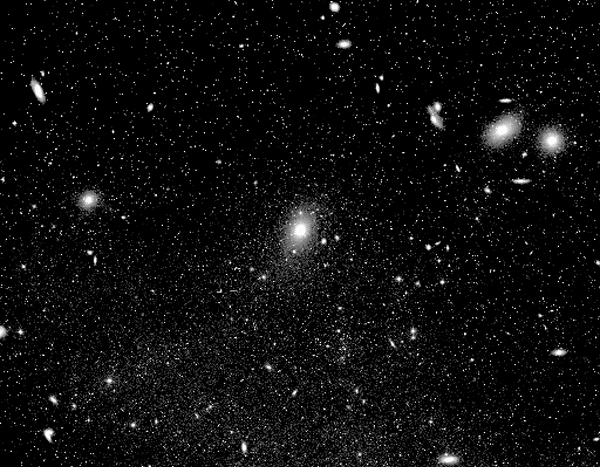## Superclusters

• This map shows that galaxy clusters are arranged in arclike superclusters.
• Size of largest superclusters = 108 ly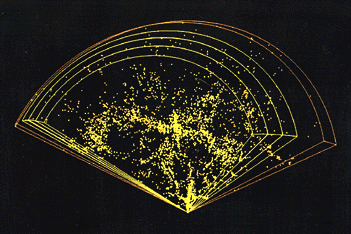## The Hubble Deep Field

• These galaxies are the most distant objects which have been observed in the Universe.
• Typical distance = 1010 ly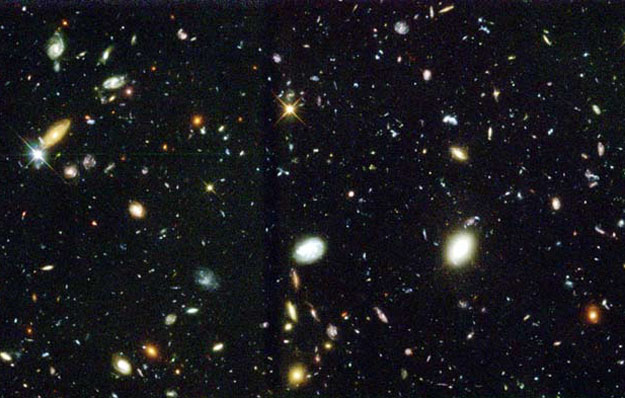Next Lecture: Angles
Read Chapter 1: 8th ed pages 8 - 15 or abridged 3rd Ed. pages 6 - 15